Courses

# Integrated Rate Law Expression Of Zero Order - Chemical Kinetics Chemistry Notes | EduRev

## Chemistry : Integrated Rate Law Expression Of Zero Order - Chemical Kinetics Chemistry Notes | EduRev

The document Integrated Rate Law Expression Of Zero Order - Chemical Kinetics Chemistry Notes | EduRev is a part of the Chemistry Course Physical Chemistry.
All you need of Chemistry at this link: Chemistry

Integrated Rate Law Expressions

Integrated rate law expression provides the predicted temporal evolution in reactant and product concentrations for reactions having an assumed order dependence.

Zero-Order Reaction

• The rate of reaction is independent of the concentration of the reactants in these reactions.
• A change in the concentration of the reactants has no effect on the speed of the reaction
• Examples of these types of reactions include the enzyme-catalyzed oxidation of CH3CH2OH (ethanol) to CH3CHO (acetaldehyde).

Consider the following elementary reaction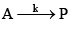For zero-order reaction, the rate law is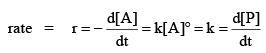k is rate constant.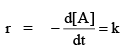⇒ –d[A] = k dt

If at t = 0, the initial concentration is [A]0 and the concentration at t = t, is [A], then integration yields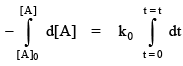⇒ [A]0 – [A] = kt
This is an integrated rate equation for a zero-order reaction in terms of reactant.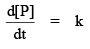= k d[P] = k dt
at              t = 0,  [P] = 0
and at        t = t, [P] = [P]
then integration yields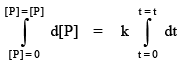[P] = kt
This is an integrated rate law equation for a zero-order reaction in terms of product.
i.e. [A]0 – [A] = kt = [P]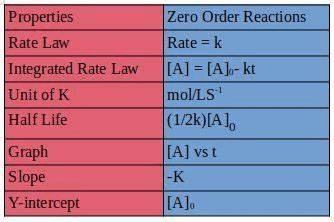Graphical Representation of Zero-Order Reaction

Graph of Reactant vs Time.

[A]0 – [A] = kt
[A] = –kt + [A]0
y = mx + c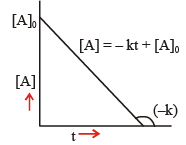Graph of Product vs Time.

[P] = kt

y = mx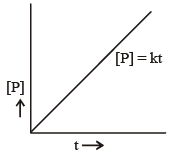Concentration of Product vs Time.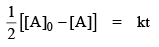[A]0 – [A] = 2kt …(i)

When t = 0 then [P] = 0 and t = t then [P] = [P]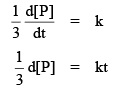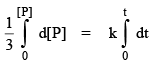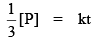[P] = 3kt     …(ii)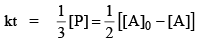…(iii)

Problem. Find the integrated rate law expression for an elementary zero-order reaction given below.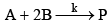Sol.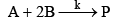The rate law of the above elementary reaction is given below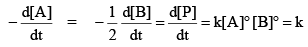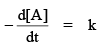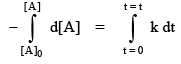⇒  – [[A] – [A]0] = kt
[A]0 – [A] = kt    …(i)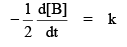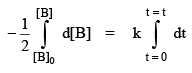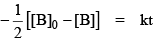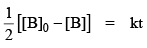…(ii)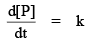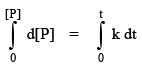[P] = kt      …(iii)

From equation (i), (ii) & (iii) we get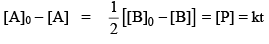Offer running on EduRev: Apply code STAYHOME200 to get INR 200 off on our premium plan EduRev Infinity!

## Physical Chemistry

84 videos|106 docs|31 tests

,

,

,

,

,

,

,

,

,

,

,

,

,

,

,

,

,

,

,

,

,

;